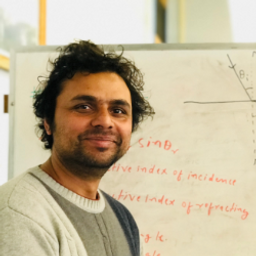## a. \text { farsighted }b. 18.0 \mathrm{cm}c. 38.0 \mathrm{cm}d. \begin{array}{l}{\text { virtual }} \\ {\text { negative }}\end{array}e. 34.2 \mathrm{cm}f. +2.92 \text { diopter } sg. \begin{array}{l}{f=40.0 \mathrm{cm}} \\ {D=+2.5 \text { diopters }}\end{array}

#### Topics

Electromagnetic Waves

Wave Optics

### Discussion

You must be signed in to discuss.
##### Top Physics 103 Educators##### Andy C.

University of Michigan - Ann Arbor##### Jared E.

University of Winnipeg### Video Transcript

In this problem, a person cannot see an object closer than 40 senator, so his near point is at 47 better, and he wants to be able to see the object from the object distance of 20 centimeter. So for part eight, to answer this question is pretty straightforward, that the person is far sighted because he cannot see the objects closer than 40 senator. So he is far sighted. Okay, for Part B, it is also given that the eye to the lens distances choose in Demeter, Therefore, with the corrective lance two centimeter in front of the eye, the object distances D O. Because 20 centimeter, minus two centimeter, it calls 18 senator, right? And for Parsi, since the near point is that four centimeter, the upright and virtual image should form at the near point when using the corrective lis. Now, since the lenses to send emir in front of the eye, the immense distance of the lens is going to be Q. We're gonna write down the magnitude of the minister's Q. Is going to be fourty centimeter minus two centimeter, and this is 38 centimeter, all right, and for party, the image must be formed in front of the lens. Therefore, the images partial you made is virtual, and but immense distance is negative. Okay, so they miss distance. Q. Is actually negative. 38 centimeter for Part E. We just used the lens equation. And if you use the lens equation for Golding can be returned in terms of P and Q object and in existence, it is going to be p times Q over P plus que and this is 18 centimeter times negative, 38 centimeter over 18 centimeter, minus 30 a centimeter. And this will give us 34 point to send emitter. I'm gonna write this down in transit meter zero point 342 meters. So for part F, we're gonna find the power of the let's, which is pee. He calls one over asked emitter, which is going to be won over 0.3 for two. And this is going to be positive. 2.92 dieters okay, now for party. If we used conduct lands instead, the distance between the lens and the I would be zero, and we would have actually the object instance p that would be 20 centimeter. Q. Would be negative for this interview. Er now, using this to the focal length, F would be p that cube overpay plus que. And this would give us 20 centimeter times 40 centimeter negative 40 cent imager over 20 centimeter, minus 40 centimeter. And this will give us 40 sand emitter or 0.4 meter. And the power of the lens in this case would be P. I'm gonna write down Capital P, which is going to be won over half in meters, huh? And one of our 0.4 would give us positive 2.5 doctors.University of Wisconsin - Milwaukee

#### Topics

Electromagnetic Waves

Wave Optics

##### Top Physics 103 Educators##### Andy C.

University of Michigan - Ann Arbor##### Jared E.

University of Winnipeg